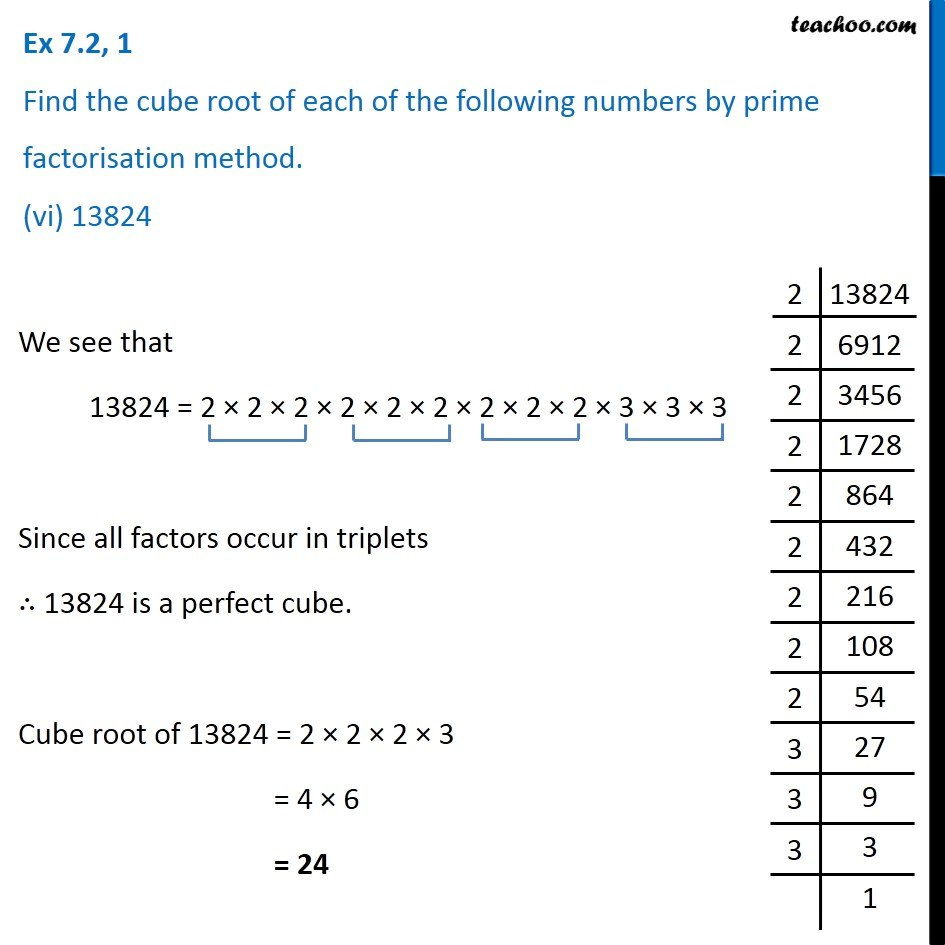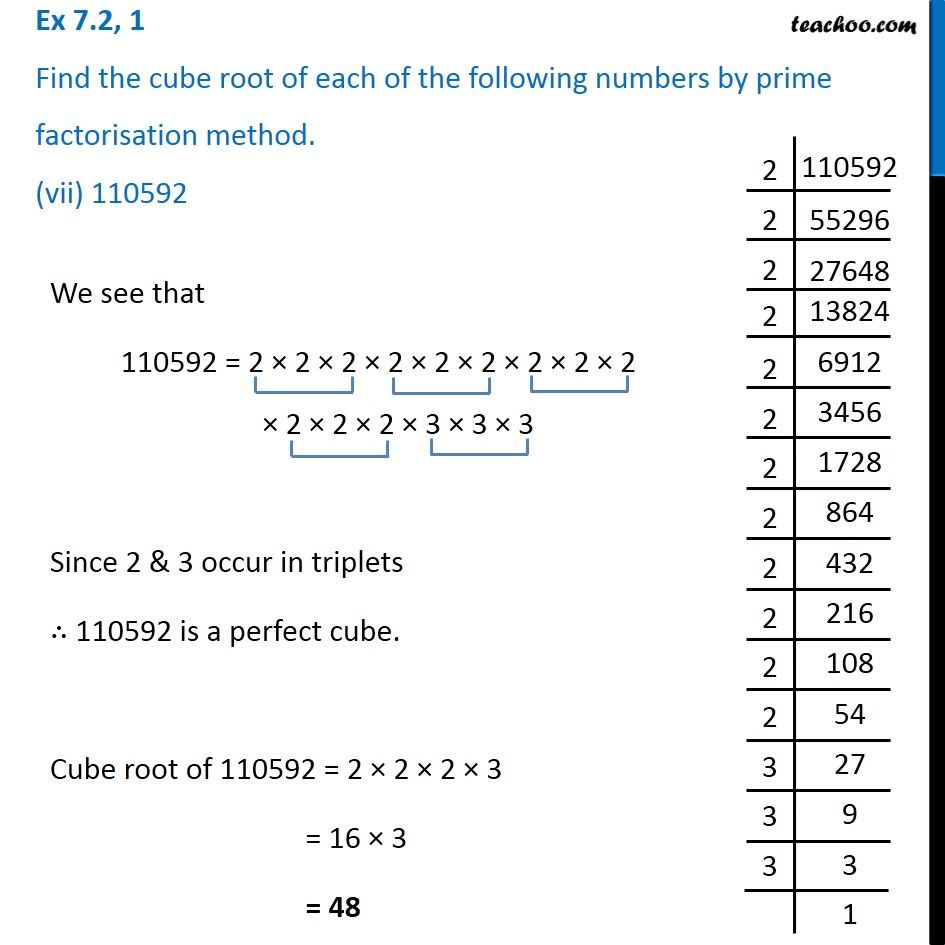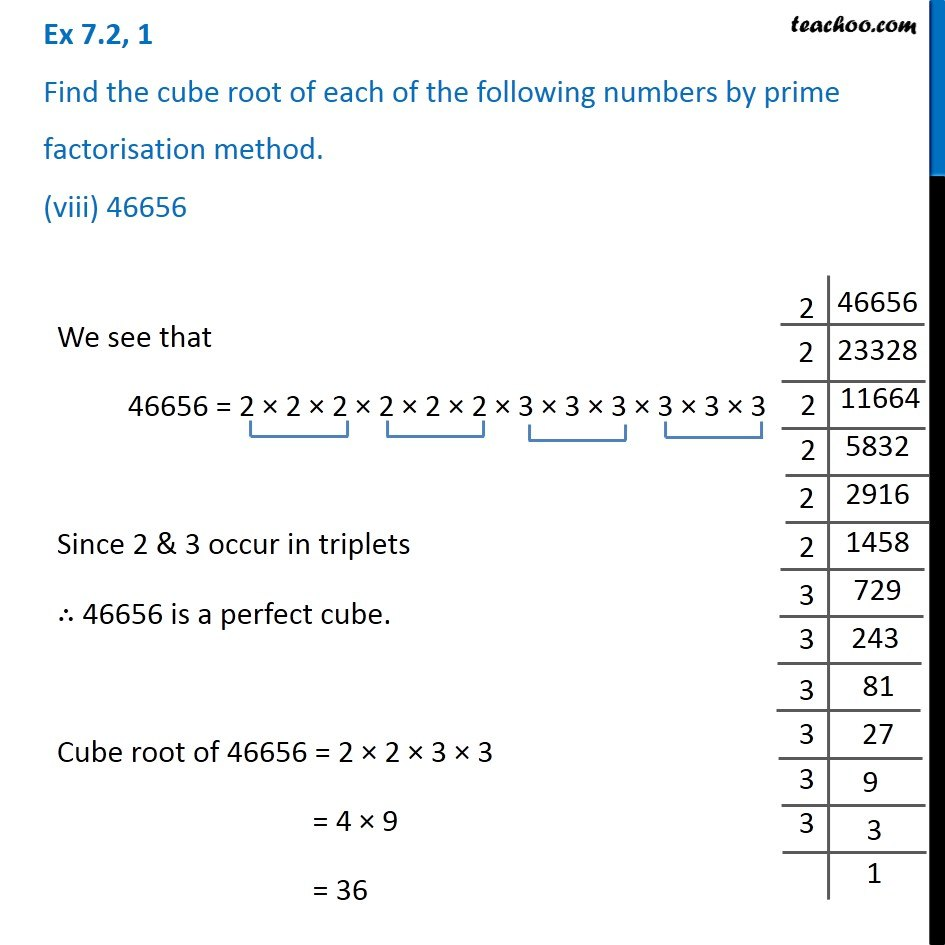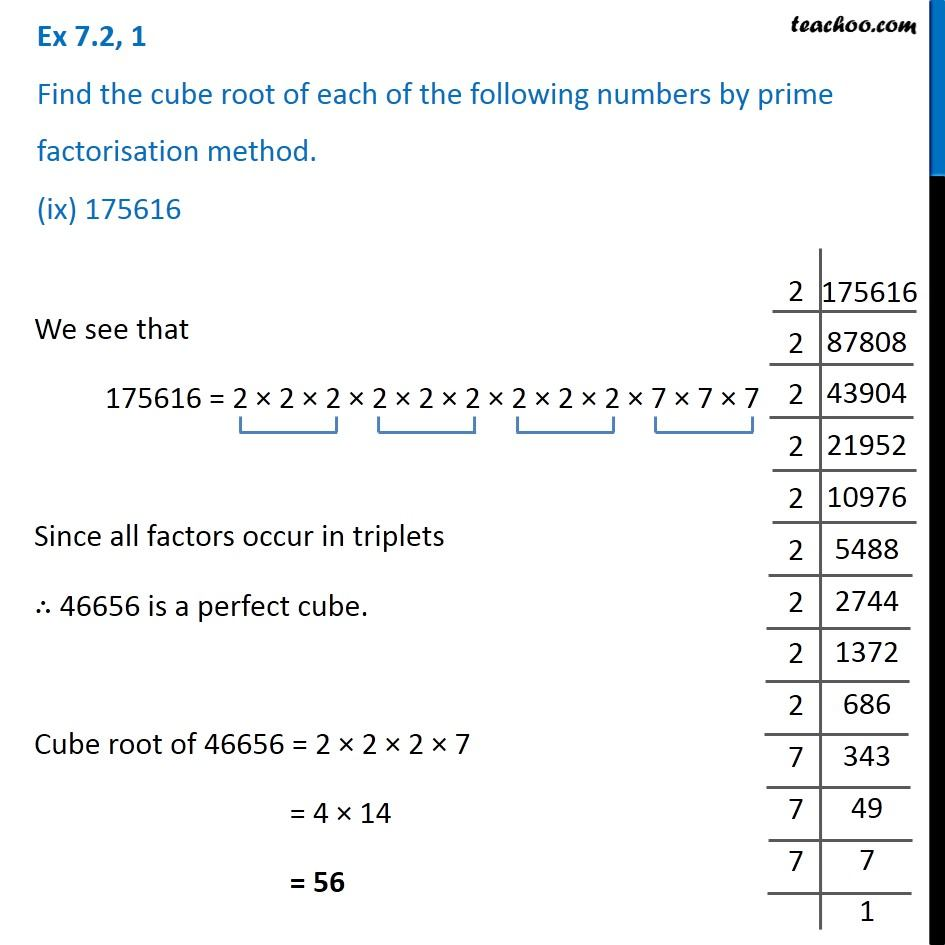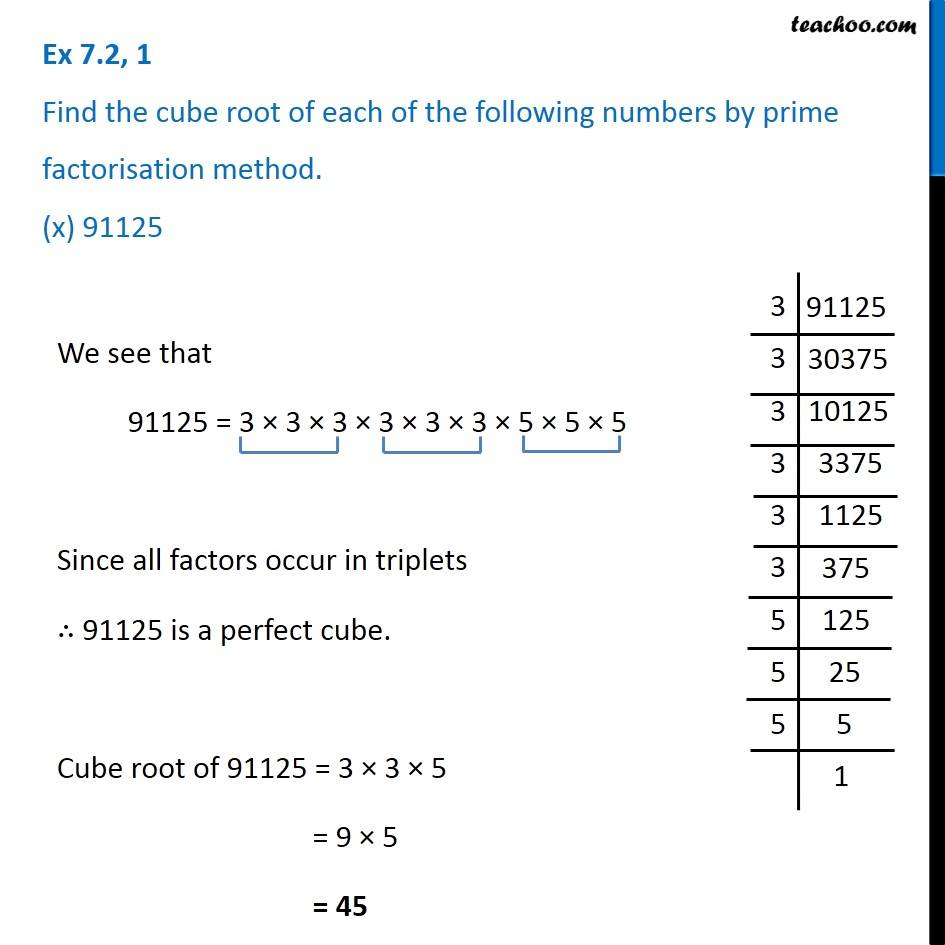1. Chapter 7 Class 8 Cubes and Cube Roots
2. Concept wise
3. Finding cube root by prime factorisation method

Transcript

Ex 7.2, 1 Find the cube root of each of the following numbers by prime factorisation method. (vi) 13824We see that 13824 = 2 × 2 × 2 × 2 × 2 × 2 × 2 × 2 × 2 × 3 × 3 × 3 Since all factors occur in triplets ∴ 13824 is a perfect cube. Cube root of 13824 = 2 × 2 × 2 × 3 = 4 × 6 = 24 Ex 7.2, 1 Find the cube root of each of the following numbers by prime factorisation method. (vii) 110592We see that 110592 = 2 × 2 × 2 × 2 × 2 × 2 × 2 × 2 × 2 × 2 × 2 × 2 × 3 × 3 × 3 Since 2 & 3 occur in triplets ∴ 110592 is a perfect cube. Cube root of 110592 = 2 × 2 × 2 × 3 = 16 × 3 = 48 Ex 7.2, 1 Find the cube root of each of the following numbers by prime factorisation method. (viii) 46656We see that 46656 = 2 × 2 × 2 × 2 × 2 × 2 × 3 × 3 × 3 × 3 × 3 × 3 Since 2 & 3 occur in triplets ∴ 46656 is a perfect cube. Cube root of 46656 = 2 × 2 × 3 × 3 = 4 × 9 = 36 Ex 7.2, 1 Find the cube root of each of the following numbers by prime factorisation method. (ix) 175616We see that 175616 = 2 × 2 × 2 × 2 × 2 × 2 × 2 × 2 × 2 × 7 × 7 × 7 Since all factors occur in triplets ∴ 46656 is a perfect cube. Cube root of 46656 = 2 × 2 × 2 × 7 = 4 × 14 = 56 Ex 7.2, 1 Find the cube root of each of the following numbers by prime factorisation method. (x) 91125We see that 91125 = 3 × 3 × 3 × 3 × 3 × 3 × 5 × 5 × 5 Since all factors occur in triplets ∴ 91125 is a perfect cube. Cube root of 91125 = 3 × 3 × 5 = 9 × 5 = 45

Finding cube root by prime factorisation method